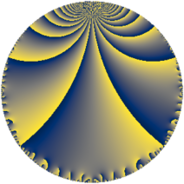# Properties

 Label 19.5.fLevel $19$ Weight $5$ Character orbit 19.f Rep. character $\chi_{19}(2,\cdot)$ Character field $\Q(\zeta_{18})$ Dimension $36$ Newform subspaces $1$ Sturm bound $8$ Trace bound $0$

# Related objects

## Defining parameters

 Level: $$N$$ $$=$$ $$19$$ Weight: $$k$$ $$=$$ $$5$$ Character orbit: $$[\chi]$$ $$=$$ 19.f (of order $$18$$ and degree $$6$$) Character conductor: $$\operatorname{cond}(\chi)$$ $$=$$ $$19$$ Character field: $$\Q(\zeta_{18})$$ Newform subspaces: $$1$$ Sturm bound: $$8$$ Trace bound: $$0$$

## Dimensions

The following table gives the dimensions of various subspaces of $$M_{5}(19, [\chi])$$.

Total New Old
Modular forms 48 48 0
Cusp forms 36 36 0
Eisenstein series 12 12 0

## Trace form

 $$36q - 6q^{2} - 18q^{3} - 48q^{4} - 6q^{5} - 48q^{7} - 9q^{8} + 246q^{9} + O(q^{10})$$ $$36q - 6q^{2} - 18q^{3} - 48q^{4} - 6q^{5} - 48q^{7} - 9q^{8} + 246q^{9} - 153q^{10} - 48q^{11} + 855q^{12} + 555q^{13} - 399q^{14} - 1647q^{15} - 2112q^{16} + 39q^{17} - 12q^{19} + 2886q^{20} + 912q^{21} + 1386q^{22} + 1074q^{23} + 168q^{24} + 2448q^{25} - 3675q^{26} + 1215q^{27} + 2316q^{28} - 1743q^{29} + 2580q^{30} - 2817q^{31} - 6039q^{32} - 7368q^{33} - 8460q^{34} - 1326q^{35} - 4581q^{36} + 2892q^{38} + 7164q^{39} + 4482q^{40} + 6150q^{41} + 22299q^{42} + 2091q^{43} + 34053q^{44} + 3165q^{45} + 7326q^{46} + 4539q^{47} - 44943q^{48} + 102q^{49} - 51876q^{50} - 25836q^{51} - 3279q^{52} - 11607q^{53} + 6069q^{54} - 7044q^{55} + 2532q^{57} + 13644q^{58} - 681q^{59} + 63654q^{60} + 44346q^{61} + 14796q^{62} + 53388q^{63} + 939q^{64} + 3636q^{65} + 11148q^{66} - 52089q^{67} + 5262q^{68} - 54954q^{69} - 69999q^{70} - 24504q^{71} - 101118q^{72} - 6666q^{73} - 9675q^{74} - 6462q^{76} + 48918q^{77} + 56871q^{78} + 41043q^{79} + 65649q^{80} + 2919q^{81} + 114711q^{82} - 17598q^{83} + 11115q^{84} + 52344q^{85} - 55716q^{86} + 2121q^{87} - 69057q^{88} - 11706q^{89} - 41394q^{90} - 84249q^{91} - 88668q^{92} - 60321q^{93} + 93405q^{95} + 167166q^{96} + 4401q^{97} + 69381q^{98} + 58386q^{99} + O(q^{100})$$

## Decomposition of $$S_{5}^{\mathrm{new}}(19, [\chi])$$ into newform subspaces

Label Dim. $$A$$ Field CM Traces $q$-expansion
$$a_2$$ $$a_3$$ $$a_5$$ $$a_7$$
19.5.f.a $$36$$ $$1.964$$ None $$-6$$ $$-18$$ $$-6$$ $$-48$$